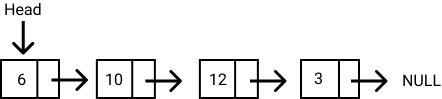# How to implement a Linked List in JavaScript

|

## What is a Linked List?

A linked list is a linear data structure similar to an array. However, unlike arrays, elements are not stored in a particular memory location or index. Rather each element is a separate object that contains a pointer or a link to the next object in that list.

Each element(which are commonly called nodes) contains two items: the data stored and a link to the next node. The data can be any valid data type. You can see this illustrated in the diagram below.The entry point to a linked list is called the head. The head is a reference to the first node in the linked list. The last node on the list points to null. If a list is empty, the head is a null reference.

In JavaScript, a linked list looks like this:

``````const list = {
value: 6
next: {
value: 10
next: {
value: 12
next: {
value: 3
next: null
}
}
}
}
}
};``````

• Nodes can easily be removed or added from a linked list without reorganizing the entire data structure. This is one advantage it has over arrays.

• Search operations are slow in linked lists. Unlike arrays, random access of data elements is not allowed. Nodes are accessed sequentually starting from the first node.
• It uses more memory than arrays because of the storage of the pointers.

There are three types of linked lists:

• Singly Linked Lists : Each node contains only one pointer to the next node. This is what we have been talking about so far.
• Doubly Linked Lists : Each node contains two pointers, a pointer to the next node and a pointer to the previous node.
• Circular Linked Lists : Circular linked list is a variation of a linked list in which the last node points to the first node or any other node before it thereby forming a loop.

## Implementing a List Node in JavaScript

As stated earlier, a list node contains two items: the data and the pointer to the next node. We can implement a list node in JavaScript as follows:

``````class ListNode {
constructor(data) {
this.data = data
this.next = null
}
}``````

## Implementing a Linked List in JavaScript

The code below shows the implementation of a linked list class with a constructor. Notice that if the head node is not passed, the head is initialized to null.

``````class LinkedList {
}
}``````

## Putting it all together

Let's create a linked list with the class we just created. First, we create two list nodes, `node1` and `node2` and a pointer from node 1 to node 2.

``````let node1 = new ListNode(2)
let node2 = new ListNode(5)
node1.next = node2``````

Next, we'll create a Linked list with the `node1`.

``let list = new LinkedList(node1)``

Let's try to access the nodes in the list we just created.

``console.log(list.head.next.data) //returns 5``

Next up, we will implement four helper methods for the linked list. They are:

1. size()
2. clear()
3. getLast()
4. getFirst()

### 1. size()

This method return the number of nodes present in the linked list.

``````    size() {
let count = 0;
while (node) {
count++;
node = node.next
}
return count;
}``````

### 2. clear()

This method empties out the list.

``````    clear() {
}``````

### 3. getLast()

This method returns the last node of the linked list.

``````    getLast() {
if (lastNode) {
while (lastNode.next) {
lastNode = lastNode.next
}
}
return lastNode
}``````

### 4. getFirst()

This method returns the first node of the linked list.

``````    getFirst() {